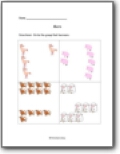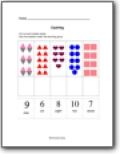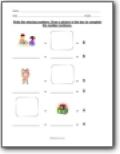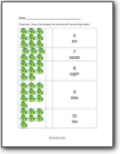# Learning Numbers Worksheets

#### What Are Numbers?

Numbers help us assign a value for something. By designating a value, it gives that something a level of validity. In some cases that mean that it will be viewed by others as very valuable or have no significance at all. Most often we use numbers to have an idea of where something stands compared to something else. Numbers can, for all intents and purposes, be considered the letters of the language of math. You would need to consider the symbols of math as their form of punctuation. One thing that can bond all spoken and written languages is math. In fact, leading scientist believe that if we were ever were to communicate with extraterrestrial life, it would be through the language of math. In this section of our website, we will explore these letters of math and how we can learn to manipulate them and put them to use for us.###### Comparing Numbers

Students will visualize the concept of more and less. This section serves as a great introduction to sequences.###### Counting

Basic counting and grouping of simple numbers found with in sets of values.###### Kindergarten and Pre-K Math

My first math lessons. This is where to begin the subject of math with students. Great Pre-K or K starters.###### Recognition of Numbers

Students learn how see a number or group of objects and assign a value to them. We also learn how to write and say our numbers.